Definition of the logarithm function as an integral

We define a function log x to be the area under the curve 1/x between 1 and x if x >= 1,and the negative of the area under the curve 1/x between 1 and x if 0 < x < 1.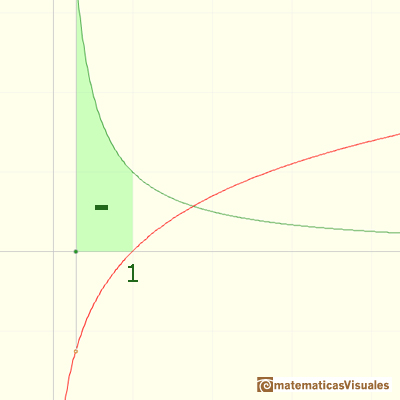In particular,

Thus log x < 0 if 0 < x < 1 (the graph lies below the x-axis) and log x > 0 if x > 1 (the graph lies above the x-axis).

You can use integral notation: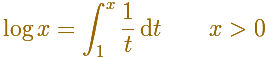This function is called the Natural Logarithm or Napierian Logarithm (in honor of their inventor, John Napier 1550-1617, although Napier follow a different approach). In some books and calculators, it is a common practice to use the symbol ln(x).

Remember that of all the integrals of power functions the integral with exponent -1 was the only one which we cannot evaluate.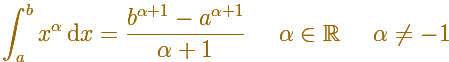The integral of power functions was know by Cavalieri from n=1 to n=9. Fermat was able to solve this problem using geometric progressions.

The Fundamental Theorem of Calculus in this case says thatThe Fundamental Theorem of Calculus tell us that every continuous function has an antiderivative and shows how to construct one using the integral.

(You can see a basic and nice demonstration of this property in Serge Lang, p. 176).If you change x (you are modifying the upper limit of integration) you can see the tangent to the logarithm function in this point and how the derivative in this point is its inverse (then the value is on the equilateral hyperbola). [The derivative is about as simple as one could ask! Spivak]

A very important property we want logarithms to have is that the logarithm of a product should be the sum of the logarithms of the individual factors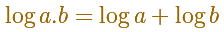We can deduce this property using the chain rule (see Serge Lang, p. 177, or Spivak, p. 315, for example). And Serge Lang wrote: "Please appreciate the elegance and efficiency of the arguments!".

But we are going to see that a property of the integral of the equilateral hyperbola justify the property of the logarithm function.

[This relation between the logarithm and the rectangular hyperbola xy=1 was discovered by the Belgian Jesuit Gregory St. Vincent in 1647]

The function log is clearly increasing (we defined it as an area). It has a positive derivative everywhere so it is strictly increasing.For small values of x, the slope is large, and moreover, it increases without bound as x decreases towards zero.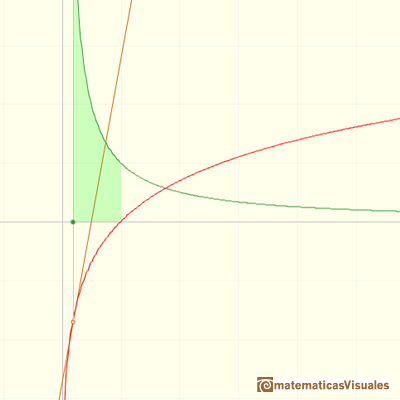The curve has slope 1 when x=1. For x > 1, the slope gradually decreases toward zero as x increases indefinitely.Since the derivative becomes very small as x becomes large, log consequently grows more and more slowly.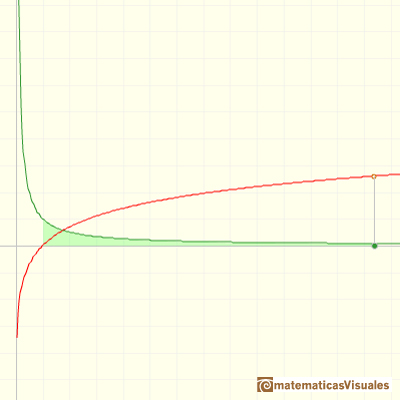It is not clear if log is bounded or not. It might be suspected that the values of log have and upper bound but you can prove that log is unbounded above nor below. Since log is continuous, it actually takes on all values. More than that, for every real number a there is one positive real number r whose logarithm is a.

Then we can define an inverse function. The domain of this inverse function is all real numbers. This important function is the Exponential function.

There is exactly one number whose natural logarithm is equal to 1. This is a very importan number, like pi, and we use a special symbol due to Leonard Euler (1707-1783), e. He was the first to recognize the importance of this number.REFERENCES

A. I. Markushevich, Areas and Logarithms, D.C. Heath and Company, 1963.
Serge Lang, A First Course in Calculus, Third Edition, Addison-Wesley Publishing Company.
Tom M. Apostol, Calculus, Second Edition, John Willey and Sons, Inc.
Michael Spivak, Calculus, Third Edition, Publish-or-Perish, Inc.
Otto Toeplitz, The Calculus, a genetic approach, The University of Chicago Press, 1963.
Kenneth A. Ross, Elementary Analysis: The Theory of Calculus, Springer-Verlag New York Inc., 1980.
C.H. Edwards, Jr., The Historical Development of the Calculus, Springer-Verlag New York Inc., 1979.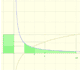The main property of a logarithm function is that the logarithm of a product is the sum of the logarithms of the individual factors.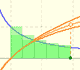The logarithm of the number e is equal to 1. Using this definition of the number e we can approximate its value.Constant e is the number whose natural logarithm is 1. It can be defined as a limit of a sequence related with the compound interest. Both definitions for e are equivalent.After the definition of the natural logarithm function as an integral you can define the exponential function as the inverse function of the logarithm.Different hyperbolas allow us to define different logarithms functions and their inversas, exponentials functions.Mercator published his famous series for the Logarithm Function in 1668. Euler discovered a practical series to calculate.By increasing the degree, Taylor polynomial approximates the exponential function more and more.The Complex Exponential Function extends the Real Exponential Function to the complex plane.The complex exponential function is periodic. His power series converges everywhere in the complex plane.The Fundamental Theorem of Calculus tell us that every continuous function has an antiderivative and shows how to construct one using the integral.The Second Fundamental Theorem of Calculus is a powerful tool for evaluating definite integral (if we know an antiderivative of the function).As an introduction to Piecewise Linear Functions we study linear functions restricted to an open interval: their graphs are like segments.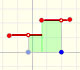A piecewise function is a function that is defined by several subfunctions. If each piece is a constant function then the piecewise function is called Piecewise constant function or Step function.A continuous piecewise linear function is defined by several segments or rays connected, without jumps between them.The integral of power functions was know by Cavalieri from n=1 to n=9. Fermat was able to solve this problem using geometric progressions.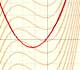If the derivative of F(x) is f(x), then we say that an indefinite integral of f(x) with respect to x is F(x). We also say that F is an antiderivative or a primitive function of f.The integral concept is associate to the concept of area. We began considering the area limited by the graph of a function and the x-axis between two vertical lines.Monotonic functions in a closed interval are integrable. In these cases we can bound the error we make when approximating the integral using rectangles.If we consider the lower limit of integration a as fixed and if we can calculate the integral for different values of the upper limit of integration b then we can define a new function: an indefinite integral of f.We can consider the polynomial function that passes through a series of points of the plane. This is an interpolation problem that is solved here using the Lagrange interpolating polynomial.